# Electronics and Communication Engineering - Signals and Systems

Exercise : Signals and Systems - Section 8
1.
The discrete time system describes by y(n) = x(n2) is
casual, Linear, time varying
casual, non-linear, timevari
non-casual, Linear, time invariant
non-casual, non-linear, time variant
Explanation:
No answer description is available. Let's discuss.

2.
If Laplace transform of f(t) is F(s), then £[tf(t)] =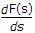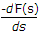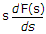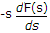Explanation:
No answer description is available. Let's discuss.

3.
A system is stable if ROC
include the unit circle
exclude the unit circle
lies on Circle
entire plane
Explanation:
No answer description is available. Let's discuss.

4.
The Laplace transform of a unit step function is
1
1 / s
s
1 / s2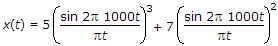would be